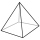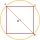Is right?

Is triangle with sides 51, 56 and 77 right triangle?

Result

Solution:Try calculation via our triangle calculator.

Leave us a comment of this math problem and its solution (i.e. if it is still somewhat unclear...):Be the first to comment!To solve this verbal math problem are needed these knowledge from mathematics:

Pythagorean theorem is the base for the right triangle calculator. See also our trigonometric triangle calculator.

Next similar examples:

1. GardenArea of a square garden is 6/4 of triangle garden with sides 56 m, 35 m, and 35 m. How many meters of fencing need to fence a square garden?
2. Axial sectionAxial section of the cone is an equilateral triangle with area 208 dm2. Calculate the volume of the cone.
3. CuboidCuboid with edge a=16 cm and body diagonal u=45 cm has volume V=11840 cm3. Calculate the length of the other edges.
4. Area of a rectangleCalculate the area of a rectangle with a diagonal of u = 12.5cm and a width of b = 3.5cm. Use the Pythagorean theorem.
5. Trapezoid MOThe rectangular trapezoid ABCD with right angle at point B, |AC| = 12, |CD| = 8, diagonals are perpendicular to each other. Calculate the perimeter and area of ​​the trapezoid.
6. Triangle SASCalculate the area and perimeter of the triangle, if the two sides are 51 cm and 110 cm long and angle them clamped is 130 °.
7. Circle chordWhat is the length d of the chord circle of diameter 36 m, if the distance from the center circle is 16 m?
8. RectangleThe rectangle is 21 cm long and 38 cm wide. Determine the radius of the circle circumscribing rectangle.
9. Tetrahedral pyramidWhat is the surface of a regular tetrahedral (four-sided) pyramid if the base edge a=7 and height v=6?
10. MovementFrom the crossing of two perpendicular roads started two cyclists (each at different road). One runs at average speed 28 km/h, the second at average speed 24 km/h. Determine the distance between them after 45 minutes cycling.
11. Base of prismThe base of the perpendicular prism is a rectangular triangle whose legs length are at a 3: 4 ratio. The height of the prism is 2cm smaller than the larger base leg. Determine the volume of the prism if its surface is 468 cm2.
12. Touch x-axisFind the equations of circles that pass through points A (-2; 4) and B (0; 2) and touch the x-axis.
13. Right ΔA right triangle has the length of one leg 7 cm and length of the hypotenuse 25 cm. Calculate the height of the triangle.
14. Trigonometric functionsIn right triangle is: ? Determine the value of s and c: ? ?
15. Right pyramidA right pyramid on a base 4 cm square has a slant edge of 6 cm. Calculate the volume of the pyramid.
16. Tree trunkWhat is the smallest diameter of a tree trunk that we can cut a square-section square with a side length of 20 cm?
17. Cube in a sphereThe cube is inscribed in a sphere with volume 3234 cm3. Determine the length of the edges of a cube.